# Vaught conjecture

(diff) ← Older revision | Latest revision (diff) | Newer revision → (diff)

Letbe a countable complete first-order theory (cf. also Logical calculus) and let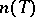be the number of countable models of, up to isomorphism (cf. also Model theory);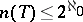. In 1961, R. Vaught [a17] asked if one can prove, without using the continuum hypothesis CH, that there is somewith. Vaught's conjecture is the statement: If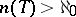, then.

Variants of this conjecture have been formulated for incomplete theories, and for sentences in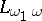. In 1970, M. Morley [a10] proved, using descriptive set theory, that if, then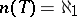or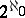(actually, he proved this for any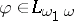).

Letbe the set of all models ofhavingas their universe (cf. also Model theory). Morley equipped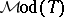with a Polish topology (cf. also Descriptive set theory). Associated with each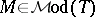is a countable ordinal number,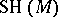, called the Scott height (or Scott rank) of. Letand, for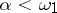, let. The isomorphism relationis analytic (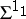; cf. also Luzin set) on; however,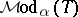is Borel (cf. also Borel system of sets) andrestricted tois a Borel equivalence relation, so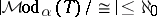or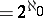. Hence (if CH fails) the only possibility forto have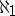countable models is that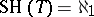and for each,.

So the Vaught conjecture may be restated as follows: If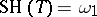, then for some,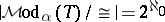. This formulation does not depend explicitly on CH.

The above Morley analysis led to the so-called topological Vaught conjecture, which is a question regarding the number of orbits of a Polish topological group (cf. also Topological group)acting in a Borel way on a Polish space[a1], [a6].

Vaught's conjecture was proved for theories of trees [a16], unary function [a7], [a9], varieties [a5], o-minimal theories [a8], and theories of modules over certain rings [a14].

In stable model theory, the combinatorial tools (like forking, cf. also Forking) developed by S. Shelah in [a4] enabled him to prove the Vaught conjecture for-stable theories [a15], which are at the lowest level of the stability hierarchy. Regarding superstable theories (the next level of the hierarchy), Vaught's conjecture was proved for weakly minimal theories [a3], [a11], and then for superstable theories of finite-rank [a2] and in some other cases [a12]. The proofs in these cases use advanced geometric properties of forking [a13].

How to Cite This Entry:
Vaught conjecture. Encyclopedia of Mathematics. URL: http://encyclopediaofmath.org/index.php?title=Vaught_conjecture&oldid=18041
This article was adapted from an original article by Ludomir Newelski (originator), which appeared in Encyclopedia of Mathematics - ISBN 1402006098. See original article